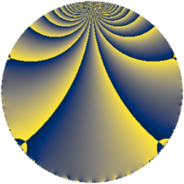# Properties

 Label 78.2.iLevel $78$ Weight $2$ Character orbit 78.i Rep. character $\chi_{78}(43,\cdot)$ Character field $\Q(\zeta_{6})$ Dimension $8$ Newform subspaces $2$ Sturm bound $28$ Trace bound $3$

# Related objects

## Defining parameters

 Level: $$N$$ $$=$$ $$78 = 2 \cdot 3 \cdot 13$$ Weight: $$k$$ $$=$$ $$2$$ Character orbit: $$[\chi]$$ $$=$$ 78.i (of order $$6$$ and degree $$2$$) Character conductor: $$\operatorname{cond}(\chi)$$ $$=$$ $$13$$ Character field: $$\Q(\zeta_{6})$$ Newform subspaces: $$2$$ Sturm bound: $$28$$ Trace bound: $$3$$ Distinguishing $$T_p$$: $$5$$

## Dimensions

The following table gives the dimensions of various subspaces of $$M_{2}(78, [\chi])$$.

Total New Old
Modular forms 36 8 28
Cusp forms 20 8 12
Eisenstein series 16 0 16

## Trace form

 $$8 q + 4 q^{4} - 4 q^{9} + O(q^{10})$$ $$8 q + 4 q^{4} - 4 q^{9} + 4 q^{10} - 4 q^{13} - 16 q^{14} - 12 q^{15} - 4 q^{16} - 8 q^{17} - 8 q^{23} + 8 q^{26} + 8 q^{29} + 4 q^{30} + 12 q^{33} + 16 q^{35} + 4 q^{36} - 12 q^{37} + 4 q^{39} + 8 q^{40} + 48 q^{41} - 4 q^{42} + 4 q^{49} + 24 q^{50} + 16 q^{51} - 8 q^{52} - 8 q^{56} - 12 q^{58} - 48 q^{59} - 12 q^{61} - 16 q^{62} - 8 q^{64} - 8 q^{65} + 24 q^{66} - 48 q^{67} + 8 q^{68} + 4 q^{69} + 24 q^{71} - 8 q^{74} + 8 q^{75} - 48 q^{77} - 16 q^{78} - 32 q^{79} - 4 q^{81} - 4 q^{82} - 12 q^{84} + 36 q^{85} - 4 q^{87} - 24 q^{89} - 8 q^{90} + 32 q^{91} - 16 q^{92} - 24 q^{93} + 24 q^{95} + 24 q^{98} + O(q^{100})$$

## Decomposition of $$S_{2}^{\mathrm{new}}(78, [\chi])$$ into newform subspaces

Label Dim $A$ Field CM Traces $q$-expansion
$a_{2}$ $a_{3}$ $a_{5}$ $a_{7}$
78.2.i.a $4$ $0.623$ $$\Q(\zeta_{12})$$ None $$0$$ $$-2$$ $$0$$ $$6$$ $$q+\zeta_{12}q^{2}+(-1+\zeta_{12}^{2})q^{3}+\zeta_{12}^{2}q^{4}+\cdots$$
78.2.i.b $4$ $0.623$ $$\Q(\zeta_{12})$$ None $$0$$ $$2$$ $$0$$ $$-6$$ $$q+\zeta_{12}q^{2}+(1-\zeta_{12}^{2})q^{3}+\zeta_{12}^{2}q^{4}+\cdots$$

## Decomposition of $$S_{2}^{\mathrm{old}}(78, [\chi])$$ into lower level spaces

$$S_{2}^{\mathrm{old}}(78, [\chi]) \cong$$ $$S_{2}^{\mathrm{new}}(13, [\chi])$$$$^{\oplus 4}$$$$\oplus$$$$S_{2}^{\mathrm{new}}(39, [\chi])$$$$^{\oplus 2}$$